# How to Count Unique Numeric Values in a Range in Excel

This post will guide you how to count unique numeric values in the given range in Excel 2013/2016 or Excel office 365. How do I count the unique numeric values in a list of data with some duplicate values using a formula in Excel. And you can use a formula that uses the SUM function together with the FREQUENCY function to count unique values in a range.

## COUNT Unique Numeric Values

Assuming you have a list of data range B1:B6 in your worksheet and you wish to count the number of unique numeric values using a formula. And the below introduction will show you the ways to achieve the result. The generic formula is like this:

=SUM(–(FREQUENCY(B1:B6,B1:B6)>0))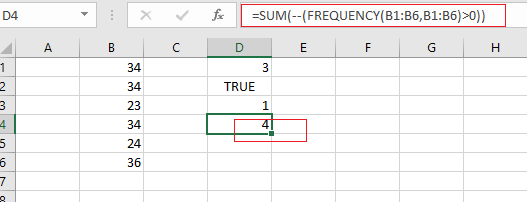Note: if you are working in Excel 365 version, and you can use a dedicated function called UNIQUE to count unique values. It can be used to count unique values including numeric or text values.

Let’s See How This Formula Works:

=FREUQENCY(B1:B6,B1:B6)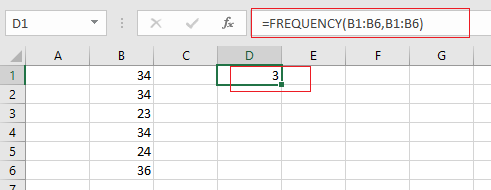The FREQUENCY function can be used to count each of numeric value in the range B1:B6, and returns an array result, like this:

{3;0;1;0;1;1;0}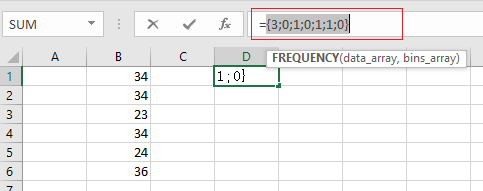From the above array result, you can see that the FREQUENCY function returns zero for any numbers that appear more that once in the data array, which is why values are zero once a number has been counted.

= FREQUENCY(B1:B6,B1:B6)>0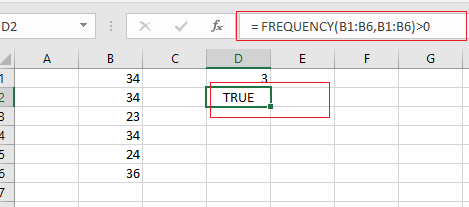You need to test each values that is greater than zero. The array result is like this:

{TRUE;FALSE;TRUE;FALSE;TRUE;TRUE;FALSE}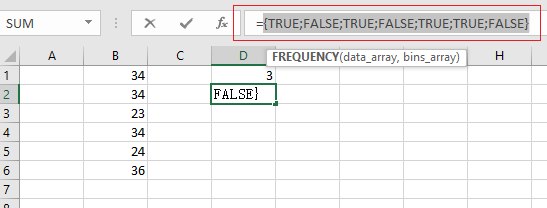Echo “TRUE” value represents a unique numeric value in the range B1:B6, and you still need to add up the TRUE values with the SUM function.

= –(FREQUENCY(B1:B6,B1:B6)>0)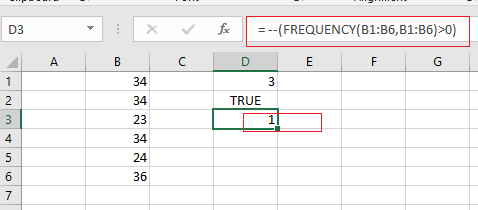The SUM function can be used to add up logic values in an array, and you need to coerce those values into 1 or 0 by the double negative operator

{1;0;1;0;1;1;0}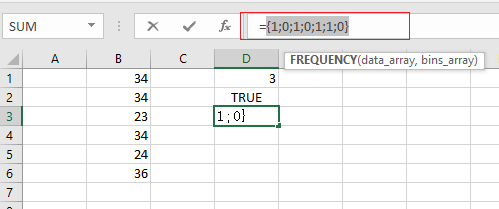Finally, you can use the SUM function to adds these values up in the above result array and return the total number is 4. Also you can use the SUMPRODUCT function to sum the values in the given array.

You can also use another way to count unique numeric values that is to use the COUNTIF function on the larger data list.

### Related Functions

• Excel SUM function
The Excel SUM function will adds all numbers in a range of cells and returns the sum of these values. You can add individual values, cell references or ranges in excel.The syntax of the SUM function is as below:= SUM(number1,[number2],…)…
• Excel FREQUENCY function
TThe Excel FREQUENCY function calculates how often values occur within a range of values. And it will return a vertical array of numbers.The syntax of the FREQUENCY function is as below:= FREQUENCY (data_array, bins_array)…
• Excel SUMPRODUCT function
The Excel SUMPRODUCT function multiplies corresponding components in the given one or more arrays or ranges, and returns the sum of those products.The syntax of the SUMPRODUCT function is as below:= SUMPRODUCT (array1,[array2],…)…
• Excel COUNTIF function
The Excel COUNTIF function will count the number of cells in a range that meet a given criteria. This function can be used to count the different kinds of cells with number, date, text values, blank, non-blanks, or containing specific characters.etc.= COUNTIF (range, criteria)…

Related Posts

Case Sensitive Lookup with SUMPRODUCT and EXACT

Today, we will show you how to use SUMPRODUCT and EXACT to perform a case sensitive exact match. In this article, we provide a simple example to calculate bonus for employees whose names are case-sensitive. If you meet similar scenarios ...

Average per Week by Formula in Excel

We usually apply AVERAGE function or relevant functions to return average directly in Excel worksheet. But in some situations, only applying average relevant functions cannot figure out our problem. Sometimes we can create a formula with functions and mathematical operation ...

How to Sum if Equal to Many Items or A Range in Excel

If we want to sum numbers from a range with criteria, we often select SUMIF of SUMIFS functions as the first choice to create a formula. The criteria can be a number or an array or a collection of some ...

How to Sum if Equal to X or Y in Excel

In daily work, if we want to sum numbers from a range, and only sum the numbers which being equal to X or Y in the range, we can create a formula with Excel build-in functions to get the result. ...

How To Sum the Largest N Values in Excel

Sometimes we may want to sum the largest N numbers or top N numbers in a range. In this article, we will show you the method of “SUM the Largest N Numbers” by a simple formula which consist of SUMPRODUCT ...

How to Sum for Cell Contains Formula Only in Excel

Sometimes values are created by formulas in cells. If we want to sum values which are created by formulas from a range, but some values which are hardcoded also list in the same range, how can we filter out matched ...

How to Sum the Smallest N Values in Excel

Sometimes we may want to sum the first smallest N numbers in a range in Excel. In this article, we will show you the method of “SUM the Smallest N Numbers” by a simple formula which consist of SUMPRODUCT and ...

How to Sum by SUMPRDUCT with One Specific Criteria Multiple Columns in Excel

Sometimes we may meet the case that to sum numbers based on one specific criteria. In this article, we will show you the method to resolve this problem by formula with the help of Excel SUMPRODUCT function. SUMPRODUCT can filter ...

How to Sum by SUMPRDUCT with Specific Criteria in Excel

Sometimes we may meet the case that to sum numbers based on two or more specific criteria. In this article, we will show you the method to resolve this problem by formulas with the help of Excel SUMPRODUCT function. SUMPRODUCT ...

How to Count Unique Dates in Excel

This post will guide you how to count unique dates in a given range in Excel 2013/2016 or Excel office 365. How do I count the unique dates in a list of dates with some duplicate dates using a formula ...

Sidebar